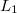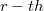﻿

### On$L_{1}$-Convergence\\ of the$r$-th Derivative of Cosine Series\\ with$r$-quasi convex coefficients

#### Abstract

We study$L_{1}$-convergence of$r-th$ derivative of modified cosine sums introduced in \cite{BN}. Exactly it is proved the$L_{1}$- convergence of$r-th$ derivative of  modified  cosine   sums with$r$-quasi convex coefficients.

DOI Code: 10.1285/i15900932v30n2p113

Keywords: cosine sums; L1-convergence; r-quasi convex null sequences

Full Text: PDF